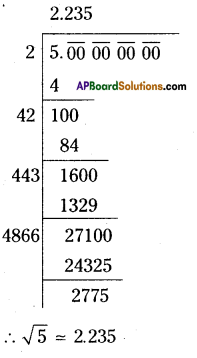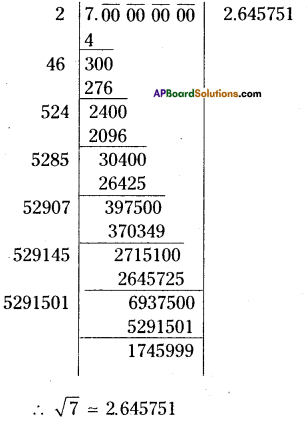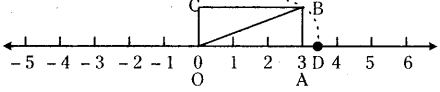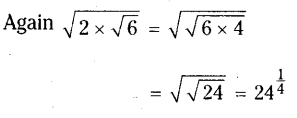AP State Syllabus AP Board 9th Class Maths Solutions Chapter 1 Real Numbers Ex 1.2 Textbook Questions and Answers.

## AP State Syllabus 9th Class Maths Solutions 1st Lesson Real Numbers Exercise 1.2

Question 1.
Classify the following numbers as rational or irrational.
i) $$\sqrt{27}$$
ii) $$\sqrt{441}$$
iii) 30.232342345
iv) 7.484848
v) 11.2132435465
vi) 0.3030030003
Solution:
i) $$\sqrt{27}$$ – irrational number
ii) $$\sqrt{441}$$ = 21 – rational
iii) 30.232342345 – irrational number
iv) 7.484848 – rational number
v) 11.2132435465 – irrational number
vi) 0.3030030003 – irrational numberQuestion 2.
Explain with an example how irrational numbers differ from rational numbers ?
Solution:
Irrational numbers can’t be expressed in $$\frac { p }{ q }$$ form where p and q are integers and q ≠ 0.
E.g.$$\sqrt{2}, \sqrt{3} ; \sqrt{5}, \sqrt{7}$$ etc.
Where as a rational can be expressed in $$\frac { p }{ q }$$ form
E.g. :- -3 = $$\frac { -3 }{ 1 }$$ and $$\frac { 5 }{ 4 }$$ etc.

Question 3.
Find an irrational number between $$\frac { 5 }{ 7 }$$ and $$\frac { 7 }{ 9 }$$. How many more there may be ?
Solution :
The decimal forms of $$\frac { 5 }{ 7 }$$ and $$\frac { 7 }{ 9 }$$ are
$$\frac{5}{7}=0 . \overline{714285} \ldots ., \frac{7}{9}=0.7777 \ldots \ldots=0 . \overline{7}$$
∴ An irrational between $$\frac { 5 }{ 7 }$$ and $$\frac { 7 }{ 9 }$$ is 0.727543…………
There are infinitely many irrational numbers between $$\frac { 5 }{ 7 }$$ and $$\frac { 7 }{ 9 }$$.

Question 4.
Find two irrational numbers between 0.7 and 0.77.
Solution:
Two irrational numbers between 0.7 and 0.77 can take the form
0.70101100111000111…………. and 0.70200200022……………

Question 5.
Find the value of √5 uPto 3 decimal places.
Solution:[√5 is not exactly equal to 2.2350679………….. as shown ¡n calculators]Question 6.
Find the value of √7 upto six decimal places by long division method.
Solution:Question 7.
Locate $$\sqrt{\mathrm{10}}$$ on number line.Step – 1 : Draw a number line.
Step – 2 : Draw a rectangle OABC at zero with measures 3 x 1. i.e., length 3 units and breadth 1 unit.
Step – 3 : Draw the diagonal OB.
Step – 4 : Draw an arc with centre ‘O’ and radius OB which cuts the number line at D.
Step – 5 : ‘D’ represents $$\sqrt{\mathrm{10}}[latex] on the number line.Question 8. Find atleast two irrational numbers between 2 and 3. Solution: An irrational number between a and b is Tab [latex]\sqrt{\mathrm{ab}}$$ unless ab is a perfect square.
∴ Irrational number between 2 and 3 is √6∴ Required irrational numbers are 61/2, 241/4

Method – II:
Irrational numbers between 2 and 3 are of the form 2.12111231234………….. and 3.13113111311113…….Question 9.
State whether the following statements are true or false. Justify your answers.
Solution:

1. Every irrational number is a real number – True (since real numbers consist of rational numbers and irrational numbers)
2. Every rational number is a real number – True (same as above)
3. Every rational number need not be a rational number – False (since all rational numbers are real numbers).
4. $$\sqrt{n}$$ is not irrational if n is a perfect square – True. (since by definition of an irrational number).
5. $$\sqrt{n}$$ is irrational if n is not a perfect square – True. (same as above)
6. All real numbers are irrational – False (since real numbers consist of rational